# What Is The Fair Price Of An Option You Buy Or Sell?

Mar. 22, 2018 5:26 PM ETSPY3 Comments
Please Note: Blog posts are not selected, edited or screened by Seeking Alpha editors.

ETF investing, Portfolio Strategy

Contributor Since 2018

Before 2014, I worked in the pension industry for 7 years being responsible for the asset management function as a Vice President on Investments of a private pension fund (around \$1 billion AuM).

Since 2014, I have been working as an investment advisor with my own private practice focusing on the long-term portfolio management with index ETFs and hedging with options.

The other part of my professional life is options trading. I have been familiar with it for the last decade, and now I trade them on a daily basis using options to build trading strategies or as a hedge for the long-term equity positions in my portfolios and portfolios of my clients.

In 2017, I decided to open to the public my software that I had been developing and using for myself for several years. I called it OptionSmie - www.optionsmile.com. It is an online analytical software platform calculating the options Fair Value on the basis of the historical distribution of the underlying security. It identifies market misprising and discovers trading opportunities in different market regimes and helps in building options trading or hedging strategies.

Since it is a by-product of my own trading that I use every day, I decided to make the access free because the incremental cost for making it public is not material for me. What is more valuable is the feedback that I’m receiving to my approach, which is somewhat unusual and is not widely used among option traders.

## Summary

• A price is fair if both the buyer and the seller have zero expected profit.
• Mathematical expectancy is a key. It equals the probability-weighted future outcomes.
• Fair Value of an option is equal to its mathematically expected payoff at expiration.
• Difference between the Fair and Market price is the expected profit of the seller of an overpriced contract (or of the buyer of an underpriced one).
• Probabilities can be derived from the historical distribution of the underlying security returns.

What does the "fair price" mean? Among many facets of "fairness", we prefer the one implying that neither party of a trade have an advantage over each other.

To be more specific, the Fair Value of a derivative security is a price at which both buyer and seller have zero expected profit.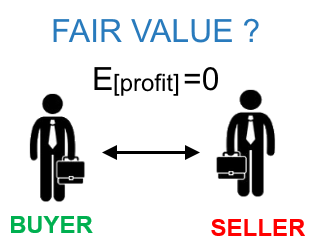When a market price exceeds this Fair Value, an option contract is overpriced and the seller has an edge over the buyer. In the opposite case, an option is supposed to be underpriced and a buyer has an expected profit.

### Seeking Fairness

Well. How can we find that magic Fair Value for an option contract?

The key term here is the "expectancy" in a mathematical sense: expected value is equal to all possible future outcomes weighted by their probabilities. Therefore, to lead to the zero expected profit, that fair option value should be equal to the expected price (payoff) at the expiration.

In short, a derivative's Fair Value is equal to its all possible values on the expiration date weighted by their probabilities: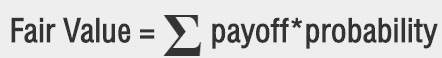Here is how it looks for a put option (X-strike, S-underlying price):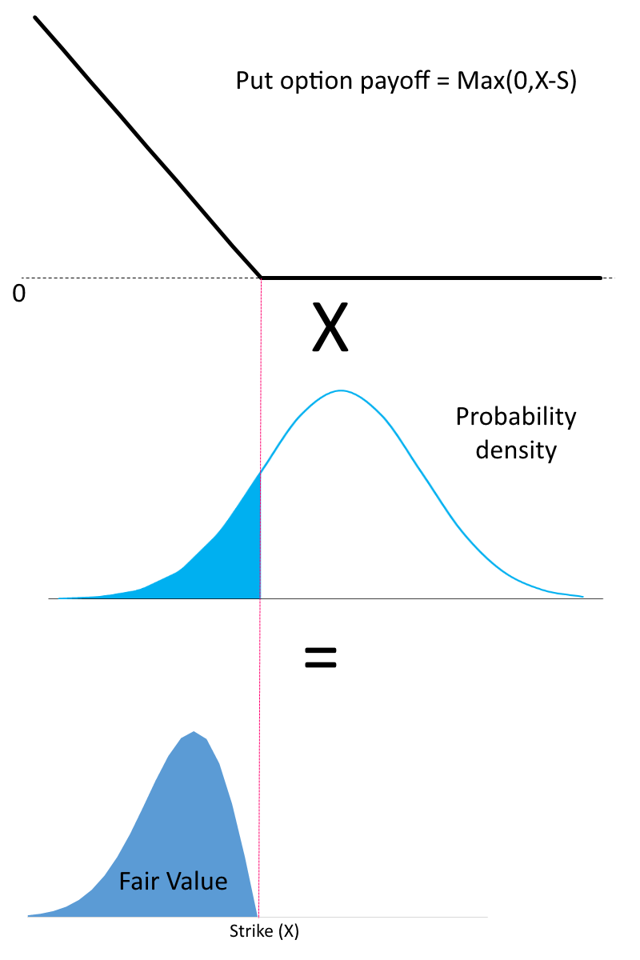The Fair Value is equal to the shaded area on the lower diagram.

Mathematically speaking, it is actually an integral of the multiplication of two functions: the payoff and the probability density.

The payoff event is expected to occur at some future point. Therefore, the final step is to calculate the present value of the Fair Value to make it comparable to the current market price. That is done with multiplication by discounting factor e-rt, where r – risk-free rate, t – time till expiration.

### Fair Value of Plain Vanilla European Options

The above formula is applicable for all types of derivative securities with a defined expiration date: futures, options, swaps, warrants, etc. Let's focus on the plain vanilla European-style options. That makes the calculation simpler thanks to the payoff function being zero in the out-of-the-money(OTM) area.

Actually, it does not matter what the probabilities are in the area when the payoff is zero. Everything multiplied by zero also equals zero.

For a put option: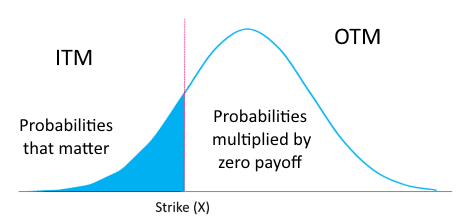Therefore, we can completely ignore the probabilities in the out-of-the-money zone and simplify the Fair Value Formula:

Fair Value = Pitm * EVitm * e-rt

Pitm – probability of the in-the-money expiration

EVitm – expected value (payoff) of an option in case of ITM expiration (conditional expectation)

e-rt – discount factor

This formula is similar to the approach used in the insurance industry. The fair (net) insurance premium is equal to the probability of an insured event - claim arrival, multiplied by the expected claim amount - claim severity.

### Putting a Probability Measure

We have found that to estimate the Fair Value and expected profit/loss we need a set of probabilities in the ITM area: on the left from the strike for puts and on the right for calls. In other words, we need to find a “probability measure” that we would assign to each possible future price (or return) of the underlying security at the options expiration.

Obviously, that is the most crucial point in the options valuation.

There are many ways to find these probabilities. The most popular one is based on the famous Black-Scholes formula assuming underlying price returns to have a log-normal probability distribution, i.e., natural logarithms of the underlying returns are normally distributed. This distribution has some standard deviation (σ) and a mean equal to the risk-free rate (r). All other parameters: time to expiration, price distance to strike (moneyness), expected dividends — are known at the moment of valuation. The key unknown parameter in this model — standard deviation — is referred by the options market as implied volatility (IV).

The drawbacks of this model are well-known and we will not stop here. Option traders know that the implied volatility serves just as a balancing parameter to compensate for all the oversimplified assumptions of the Black-Scholes model (like the normality of returns distribution, risk-free mean return, etc). That is where the volatility smile (or surface) is born.

The alternative way is not to try to find a “proper” mathematical model for the probability distribution. Why not rely on the actual historical underlying returns without fitting them into some type of probability model with a closed-form function.

That constitutes a

non-parametric (model-free) method of options valuation

which quite simple:

We select all historical returns when the underlying security actually “jumped over” the strike of an option contract and settled there at the expiration. The proportion of such “flyovers” in the whole returns dataset is an estimation of the probability of the in-the-money expiration (Pitm); their average jumping distance from the strike is an expected value (payoff) of an option in case of ITM expiration (EVitm).

Below is the real example of the valuation of a call option on SPDR S&P 500 ETF (SPY) with 103 moneyness and 2 weeks till expiration.

Here is a histogram of the actual 2-week returns distribution of SPY returns (dividends-adjusted) for some lookback period (from Jan 1, 2000 to Dec 31, 2016):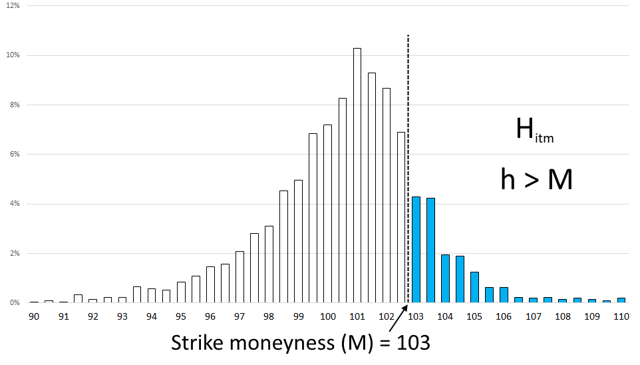Hitm is a number of returns (h) exceeding the moneyness of our contract (M). It is actually the number of hypothetical ITM-expirations in the past. In our example, it is 647 out of total 4,277 days in the dataset.

That leads to the estimation of the ITM-expiration probability: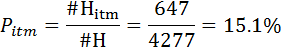In the next step, for each of the 647 ITM returns, we can easily calculate hypothetical values (payoffs) of our call option at all these expiration moments. They are simply equal to the distance of the underlying price from the strike.

By averaging 647 ITM payoffs, we get the desired expected value of an option expired ITM: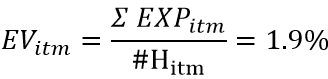This value shows how far the underlying price has been "overflying" the 103 moneyness on average in history.

Finally, we can calculate the desired Fair Value of our call option just by multiplying these two values and discounting by e-rt to get the current value of the contract (in % of the underlying price):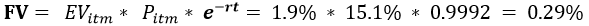As stated above, the fairness of this value means that both the seller and the buyer of an option have had zero total profit, should they have been making deals at this exact price in the past. Neither of them would have had a statistical edge. In other words, if all trades with this options contract had been made at this price (0.29% of the underlying security price), both buyer and seller would have obtained a zero total profit at the end, not taking into account the transaction costs.

In the same way, we can apply this formula for each moneyness and time to expiration and come to the Fair Value of any option contract. We even can derive the Fair Implied Volatility according to the Black-Scholes model and build the Fair Volatility Surface.

### Finding Market Mispricing

Note, we have done all these calculations without any reference to the options market. That Fair Value does not depend on views and opinions of the option market participants, and, hence, that is an “objective” option price depending only on the past behavior of the underlying security.

The final natural step is to compare our Fair Value with the options market prices.

Here is an example of the call options series on SPY where the actual Market Prices (green) are overlapping the Fair Values (blue) of the same contacts: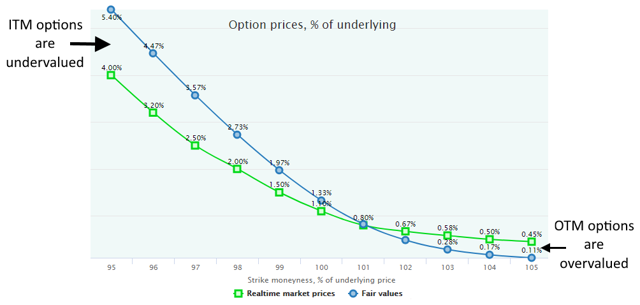The position of these two lines means that ITM (moneyness <100) call options are undervalued by the market, and a buyer of such options has a theoretical statistical advantage. The opposite is true for the OTM part (moneyness>100), where the market is ready to pay for options more than their Fair Values. In such a market disposition, the obvious trading strategy should be to buy an ITM option and sell an OTM option (debit call spread).

It should be already obvious that the difference between these two lines constitutes a mathematically expected profit/loss per trade for a buyer/seller.

Again, the key term here is “expected” that is far from “guaranteed,” of course. A seller of an overpriced contract just has a "tailwind", and not every trade will be profitable. However, the average profit per trade will converge to its expectancy after some number of trades. The same is true for a buyer of the underpriced options.

The ideas presented above are just the very basics of the foundation methodology of the OptionSmile project.

It is a free online software platform that not only finds the market mispricing but also estimates its statistical significance, filters out market regimes in the history, calculates various performance metrics for option strategies, etc.

Visit www.optionsmile.com for more information or just watch this short explainer video:

Disclosure: I am/we are long SPY.

I wrote this article myself, and it expresses my own opinions. I am not receiving compensation for it. I have no business relationship with any company whose stock is mentioned in this article.